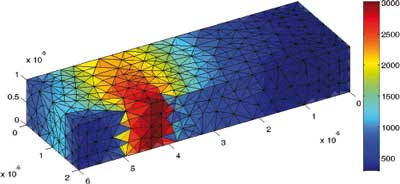## Classical Semiconductors

The classical transport of charge carrier in semiconductors can be described by the semiconductor Boltzmann equation. Often, only partial information is needed such that macroscopic models may be sufficient to predict the current flow through semiconductor devices. In industrial applications, usually the drift-diffusion model or extended models, such as the energy-transport equations, are used. For gate lengths between 25 and 100 nanometers, so-called six-moments models give very good simulation results. All these models are nonlinear partial differential equations of parabolic type.

The derivation and numerical approximation of these models offers several scientific challenges. Examples are the modeling of hot electrons, ionization, analytical treatment of the equations, and the discretization by mixed finite-element methods. The corresponding Ph.D. projects will be conducted with the participation of the following professors from electro-engineering and mathematics: Grasser, Jüngel, Melenk, Praetorius.Carrier temperature in a field-effect transistor